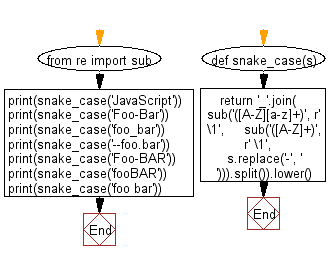﻿ Python: Convert a given string to snake case - w3resource# Python: Convert a given string to snake case

## Python String: Exercise-97 with Solution

From Wikipedia,
Snake case (stylized as snake_case) refers to the style of writing in which each space is replaced by an underscore (_) character, and the first letter of each word written in lowercase. It is a commonly used naming convention in computing, for example for variable and subroutine names, and for filenames. One study has found that readers can recognize snake case values more quickly than camel case.

Write a Python program to convert a given string to snake case.

• Use re.sub() to match all words in the string, str.lower() to lowercase them.
• Use re.sub() to replace any - characters with spaces.
• Finally, use str.join() to combine all words using - as the separator.

Sample Solution:

Python Code:

``````from re import sub
def snake_case(s):
return '_'.join(
sub('([A-Z][a-z]+)', r' \1',
sub('([A-Z]+)', r' \1',
s.replace('-', ' '))).split()).lower()

print(snake_case('JavaScript'))
print(snake_case('Foo-Bar'))
print(snake_case('foo_bar'))
print(snake_case('--foo.bar'))
print(snake_case('Foo-BAR'))
print(snake_case('fooBAR'))
print(snake_case('foo bar'))
```
```

Sample Output:

```java_script
foo_bar
foo_bar
foo.bar
foo_bar
foo_bar
foo_bar
```

Flowchart:## Visualize Python code execution:

The following tool visualize what the computer is doing step-by-step as it executes the said program:

Python Code Editor:

Have another way to solve this solution? Contribute your code (and comments) through Disqus.

What is the difficulty level of this exercise?

Test your Programming skills with w3resource's quiz.

﻿

## Python: Tips of the Day

Check if a given key already exists in a dictionary:

In is the intended way to test for the existence of a key in a dict.

```d = {"key1": 10, "key2": 23}

if "key1" in d:
print("this will execute")

if "nonexistent key" in d:
print("this will not")
```

If you wanted a default, you can always use dict.get():

```d = dict()

for i in range(100):
key = i % 10
d[key] = d.get(key, 0) + 1
```

and if you wanted to always ensure a default value for any key you can either use dict.setdefault() repeatedly or defaultdict from the collections module, like so:

```from collections import defaultdict

d = defaultdict(int)

for i in range(100):
d[i % 10] += 1
```

but in general, the in keyword is the best way to do it.

Ref: https://bit.ly/2XPMRyz